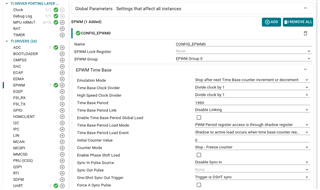If you have a related question, please click the "Ask a related question" button in the top right corner. The newly created question will be automatically linked to this question.

Part Number: LP-AM263

What is the minimum Conversion time for ADC? Does it capable to work with microseconds? The goal, is to do a new conversion every 1.1 us

• Hi Alex,

I have checked with the ADC expert, here's what I got.

ADC conversion time is 10.5* ADC_CLK  --> ADC_clk is atleast 3*sysclk --> sysclk is 200Mhz or 5ns

(sample and hold) + (conversion) --> (>=16)+(>=32) sysclks = 48 sysclks = 240nS

So, in short. yes it is possible to achieve a conversion time of 1.1uS with our ADC's.

Thanks,
G Kowshik

• Could you recommend API to use, for measure ADC value every 1.1 uS for a period of time ? (40 uS for example)
• Hi Alex,

Yes we already have an example demonstrating similar application, here's the documentation for it https://software-dl.ti.com/mcu-plus-sdk/esd/AM263X/latest/exports/docs/api_guide_am263x/EXAMPLES_DRIVERS_ADC_SOC_EPWM.htmlIn the above picture, the period is 1999 here, dividers are 1, 1 and the sysclk is TBCLK i.e., 200MHz or 5ns

Now we need a trigger at each 1.1uS. or 5ns*(220).

So program the period of epwm to be 219 -> [it will count from 0 to 219 that is 220 steps]

This triggers ADC each 1.1uS, and the ADC int happens every 1.1uS.

Hope this helps.,.

Thanks,
G Kowshik

• need to test, I'll come back